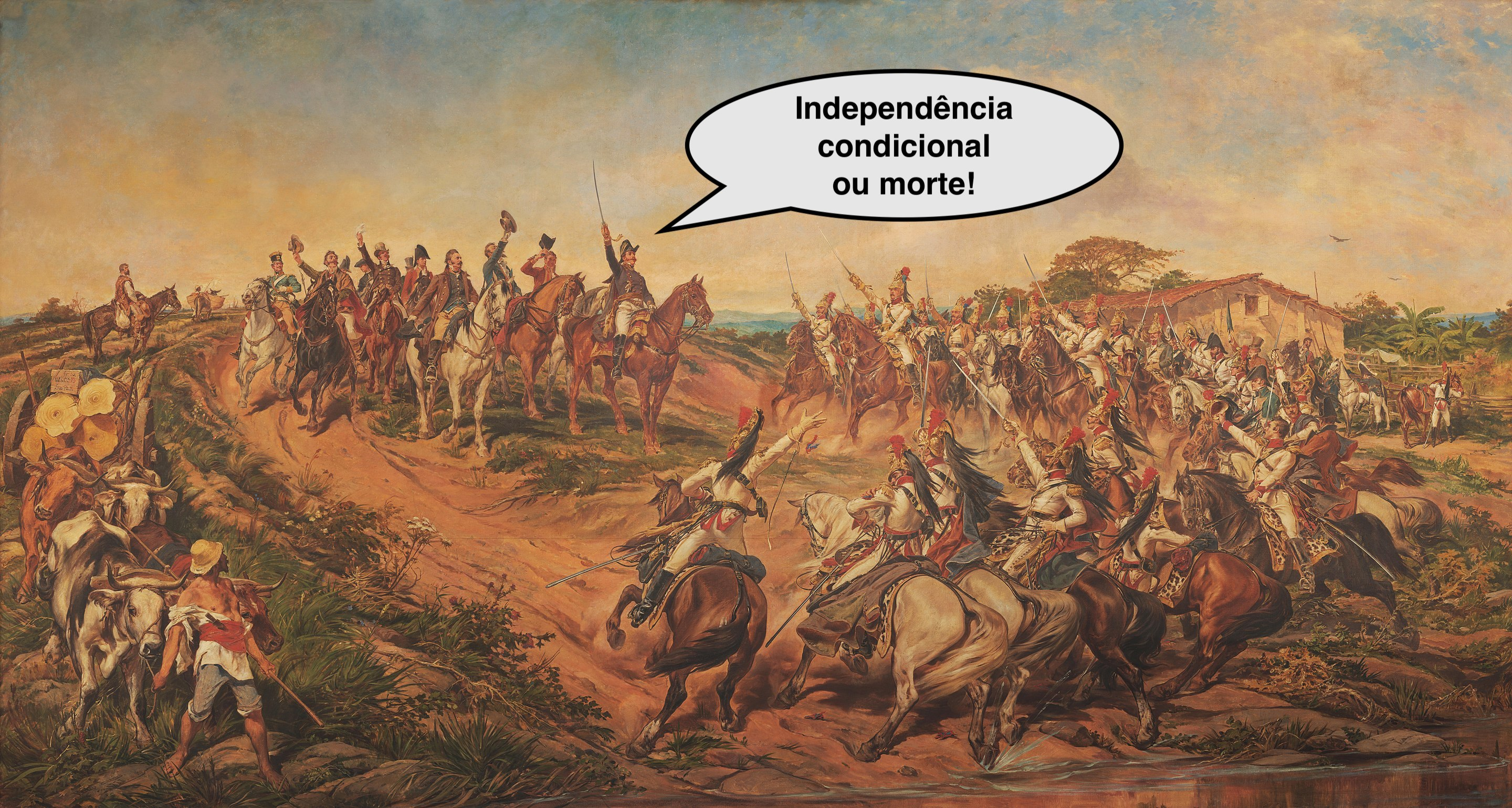## 3.4 Conditional probability

Conditional probability is the probability of event $$A$$ after observing the occurrence of an event $$B$$. The probability of $$A$$ given $$B$$ is

$\begin{equation} Pr(A|B) = \dfrac{Pr(A \cap B)}{Pr(B)}, \; \; Pr(B) \ne 0 \tag{3.32} \end{equation}$

Analogously $\begin{equation} Pr(B|A) = \dfrac{Pr(A \cap B)}{Pr(A)}, \; \; Pr(A) \ne 0 \tag{3.33} \end{equation}$

Example 3.19 (Conditional probability) A balanced die is rolled, and we wish to observe the event $$A$$: ‘side 2’. The person who threw the dice also gives information $$B$$: ‘the side is even’. Therefore, $Pr(B) = \frac{1}{2},$ $Pr(A \cap B) = \frac{1}{6},$ $Pr(A|B) = \dfrac{1/6}{1/2} = \dfrac{1}{3},$ $Pr(A^{c}|B) = 1 - \dfrac{1}{3} = \dfrac{2}{3}.$

The Equations (3.32) and (3.33) result in the chain rule , or the probability of the intersection event:

$\begin{equation} Pr(A \cap B) = Pr(A) \cdot Pr(B|A) = Pr(B) \cdot Pr(A|B) \tag{3.34} \end{equation}$

Three events $\begin{equation} Pr(A \cap B \cap C) = Pr(A) \cdot Pr(B|A) \cdot Pr(C|A \cap B) \tag{3.35} \end{equation}$

General form $\begin{equation} Pr(\cap_{i=1}^k A_i) = Pr(A_1) \cdot Pr(A_2|A_1) \cdot Pr(A_3|A_1 \cap A_2) \cdots Pr(A_k|A_1 \cap \cdots \cap A_{k-1}) \tag{3.36} \end{equation}$

Exercise 3.8 Redo Example 3.19 considering the information $$C$$: ‘the face is odd’. Calculate:

1. $$Pr(C)$$
2. $$Pr(A \cap C)$$
3. $$Pr(A \mid C)$$
4. $$Pr(A^C \mid C)$$

$$\\$$

### 3.4.1Independence

When $\begin{equation} Pr(A|B) = \dfrac{Pr(A) \cdot Pr(B)}{Pr(B)} = Pr(A) \tag{3.37} \end{equation}$ $$A$$ and $$B$$ are said to be independent, symbolized by $$A \perp\!\!\!\perp B$$. This indicates that observing $$B$$ does not change the probability with respect to $$A$$.

The probability properties still hold, allowing us to do, for example, $\begin{equation} Pr(A|B) = 1 - Pr(A^{c}|B) \tag{3.38} \end{equation}$

### 3.4.2Conditional independenceConditional Independence or Death! based on Independence or Death! by Pedro Americo (1888)

$$\\$$ Let $$A$$, $$B$$ and $$C$$ be events of $$\Omega$$. $$A$$ and $$B$$ are conditionally independent given $$C$$ if and only if

$\begin{equation} Pr(A|B,C) = Pr(A|C) \tag{3.39} \end{equation}$

For more details see and . For a cultural little moment see Paulo César Garcez Marins | Provoca | 06/06/2023.

### References

Basu, D, and Carlos AB Pereira. 2011. “Conditional Independence in Statistics.” Selected Works of Debabrata Basu, 371–84.
Pfeiffer, Paul E, and David A Schum. 1973. Introduction to Applied Probability. Elsevier.
Studený, Milan. 2005. Probabilistic Conditional Independence Structures. Springer Science & Business Media.